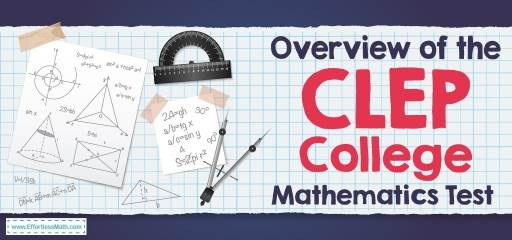# Overview of the CLEP College Mathematics TestThe College-Level Examination Program, or also known as the CLEP, is a set of 33 standardized tests that assess your knowledge in specific topics. By earning a satisfactory score on the computer-based CLEP exam, you can earn the credit of thousands of universities and colleges.

The CLEP College Mathematics defines your knowledge of the mathematical concepts taught in a university course for non-mathematical majors. It contains approximately 60 multiple choice questions that will be answered in 90 minutes. Some of these questions are pre-test questions that are not graded.

College math test questions require candidates to show the following abilities in approximate proportions shown.

• Solve routine and straightforward problems (about 50% of the examination)
• Solve unusual problems that require an understanding of concepts and the application of concepts and skills (about 50% of the examination)

## What topics does the CLEP College Mathematics cover?

The subject of the College Mathematics test is extracted from the following topics.

### Algebra and Functions (20%)

• Solving equations, linear inequalities, and systems of linear equations by graphical and analytic methods
• Interpretation, representation, and evaluation of functions: numerical, graphical, symbolic, and descriptive methods
• Graphs of functions: horizontal and vertical reflections, translations, and symmetry about the x-axis, the y-axis, and the origin
• Linear and exponential growth

### Counting and Probability (10%)

• Counting problems: the multiplication rule, permutations, and combinations
• Probability: intersection, union, independent events, mutually exclusive events, conditional probabilities, complimentary events, and expected value

### Data Analysis and Statistics (15%)

• Data interpretation and representation: tables, line graphs, bar graphs, circle graphs, pie charts, scatterplots, and histograms
• Numerical summaries of data: mean, median, mode, and range
• Standard deviation, normal distribution

### Financial Mathematics (20%)

• Percent, percent change, markups, discounts, taxes, profit, and loss
• Interest: simple, compound, continuous interest, effective annual yield, effective interest rate, or annual percentage rate
• Present value and future value

### Geometry (10%)

• Properties of triangles and quadrilaterals: area, perimeter, similarity, and the Pythagorean theorem
• Parallel and perpendicular lines
• Properties of circles: area, circumference, central angles, inscribed angles, and sectors

### Logic and Sets (15%)

• Logical operations and statements: conjunctions, conditional statements, disjunctions, negations, logical conclusions, hypotheses, converses, inverses, counterexamples, contrapositives, logical equivalence
• Set relationships, subsets, disjoint sets, Venn diagrams, and equality of sets
• Operations on sets: union, intersection, and complement

### Numbers (10%)

• Properties of numbers and their operations: rational and integers, irrational, and real numbers (including recognizing rational and irrational numbers)
• Elementary number theory: primes and composites, factors and divisibility, odd and even integers, and the fundamental theorem of arithmetic
• Measurement: scientific notation, unit conversion, and numerical precision
• Absolute value

## Is the CLEP College Mathematics hard?

The CLEP College Mathematics is not difficult, and you can pass the test with enough effort.

## Do you get a formula sheet in the CLEP College Mathematics?

The CLEP College Math test does not provide you with a formula sheet, so you need to memorize all the important formulas.

## Can you use a calculator on the CLEP College Mathematics?

You do not need to bring a calculator with you to the test center as a scientific calculator is available during the test.

## What is the passing score of CLEP College Mathematics?

The score of the test is from 20 to 80 points, and usually, a score of 50 is considered the minimum passing score.

Looking for the best resources to help you or your student succeed on the CLEP College Mathematics test?

## The Best Books to Ace the CLEP College Mathematics Test

### What people say about "Overview of the CLEP College Mathematics Test - Effortless Math: We Help Students Learn to LOVE Mathematics"?

No one replied yet.

X
49% OFF

Limited time only!

Save Over 49%

SAVE \$34

It was \$69.99 now it is \$35.99